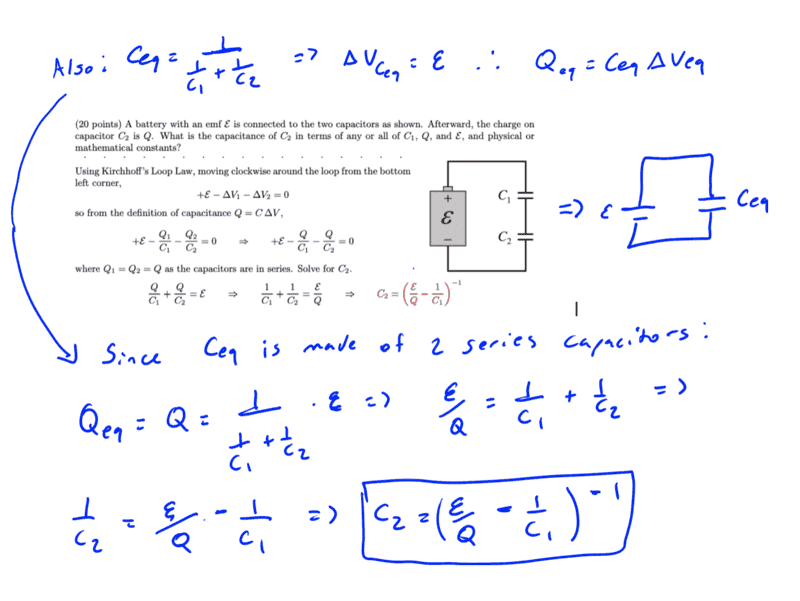# EMF and how it's related to Potential Difference

guyvsdcsniper
Homework Statement:
What is capicitance C2?
Relevant Equations:
C=Q/V
So Kirchoff's Loop law states that, The sum of all the potential differences encountered while moving around a loop or closed path is zero.
Ok so that is basically a statement of energy conservation. So I see why in the TYPED solution, they related all voltages in the circuit equal to zero. I don't understand why ΔV1 and ΔV2 are negative. Is it because moving clockwise from the bottom left corner means you are traveling up while passing the battery and down across the capacitors?

And that would imply the EMF is equal to the sum of ΔV? Is that because without the Potential Difference of the circuit comes from the EMF?